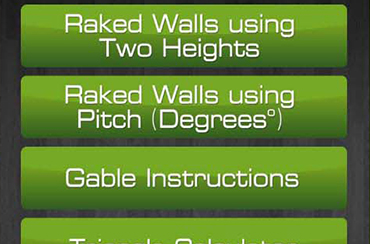This app is used to calculate measurement and required material to create wall. It eases the wall creation problem by removing mathematics using its formula. App contains different kind of formula to calculate required material and different measure- ment for wall creation.

## Functionality

• Triangle calculator
• Calculate raked walls using two height
• Calculate raked walls using pitch(degrees)

## Technology

 Platform iOS Programming Language Swift Database SQLite Tools XCode